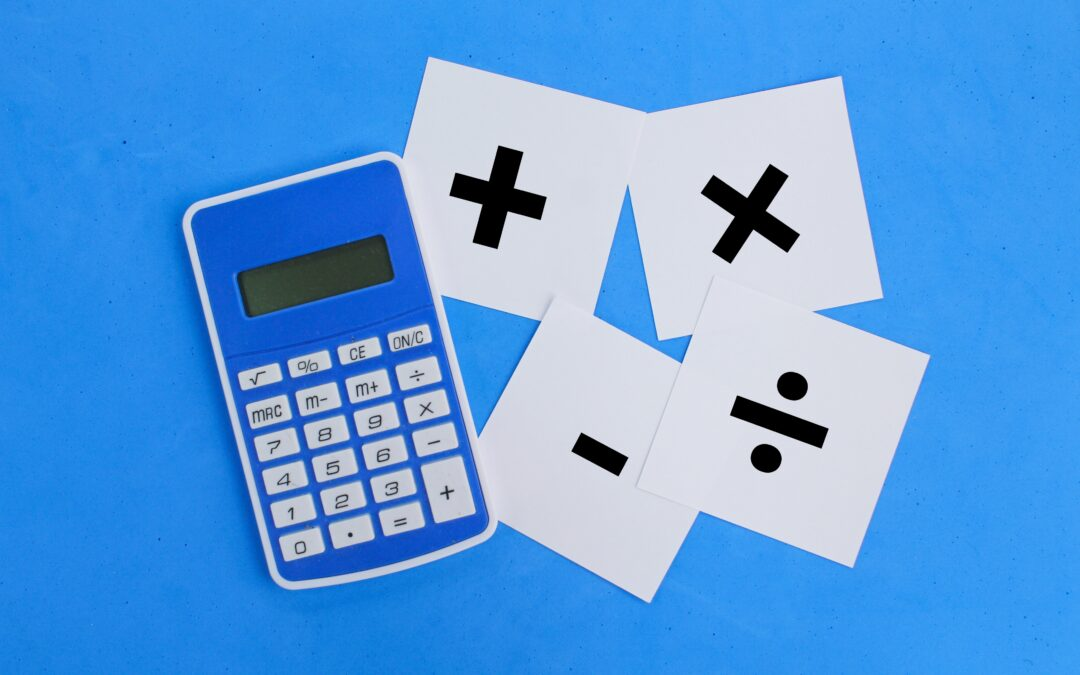Select Page## 5 Reasons to Get an Online Math Tutor

For many students, math is the most difficult subject in school. The consideration of tutoring crosses many minds of students as concepts become increasingly more difficult. When considering the choices of tutoring, the options seem endless with the two primary...## High School Math Classes | Why Students Struggle

For many students in high school, math classes pose the biggest academic obstacle. There’s a number of reasons why a student struggles with math and I want to discuss what these reasons are and why. The Why Complex Concepts One of the biggest hurdles for...## How Much is a Tutor?

As a parent, it can be overwhelming at the choices you have when it comes to tutors. How much is a tutor? Should I do in person or remote tutoring? Does it actually work? These are all valid questions to be asking yourself while considering a tutor for your student....## What is Tutoring?

So what is tutoring exactly? Here at TenneyTutors we pride ourselves on our one-to-one, online style of tutoring. Every single one of our tutors are one-to-one specialized private school teachers at our school: The Tenney School. The benefit of having a private school...## What Does Quotient Mean in Math?

Mathematics has a number of specified terminology that is used to describe certain types of numbers, equations, formulas, and more. So what does quotient mean in math? Well the quotient is the answer or result of dividing two numbers by each other. Lets look at some...## What Does Difference Mean in Math?

Mathematics can become quickly confusing as new concepts are introduced as students progress through higher and higher math courses. So what does difference mean in math? Well to put it shortly, the difference is the result of subtracting two or more separate numbers...